## Section2.2Numerical summaries and box plots

### Subsection2.2.1Measures of center

###### OpenIntro: Examining Numerical Data video

In the previous section, we saw that modes can occur anywhere in a data set. Therefore, mode is not a measure of center. We understand the term center intuitively, but quantifying what is the center can be a little more challenging. This is because there are different definitions of center. Here we will focus on the two most common: the mean and median.

The mean, sometimes called the average, is a common way to measure the center of a distribution of data. To find the mean number of characters in the 50 emails, we add up all the character counts and divide by the number of emails. For computational convenience, the number of characters is listed in the thousands and rounded to the first decimal.

\begin{gather} \bar{x} = \frac{21.7 + 7.0 + \cdots + 15.8}{50} = 11.6\label{sampleMeanEquation}\tag{2.2.1} \end{gather}

The sample mean is often labeled $\bar{x}\text{.}$ The letter $x$ is being used as a generic placeholder for the variable of interest, num_char, and the bar on the $x$ communicates that the average number of characters in the 50 emails was 11,600.

###### Mean

The sample mean of a numerical variable is computed as the sum of all of the observations divided by the number of observations:

\begin{gather} \bar{x} = \frac{1}{n}\sum{x_{i}} = \frac{x_1+x_2+\cdots+x_n}{n}\label{meanEquation}\tag{2.2.2} \end{gather}

where $\sum$ is the capital Greek letter sigma and $\sum{x_{i}}$ means take the sum of all the individual $x$ values. $x_1, x_2, \dots, x_n$ represent the $n$ observed values.

Examine Equation (2.2.1) and Equation (2.2.2) above. What does $x_1$ correspond to? And $x_2\text{?}$ What does $x_i$ represent? 1 $x_1$ corresponds to the number of characters in the first email in the sample (21.7, in thousands), $x_2$ to the number of characters in the second email (7.0, in thousands), and $x_i$ corresponds to the number of characters in the $i^{th}$ email in the data set.

What was $n$ in this sample of emails?  2 The sample size was $n=50\text{.}$

The email50 data set represents a sample from a larger population of emails that were received in January and March. We could compute a mean for this population in the same way as the sample mean, however, the population mean has a special label: $\mu\text{.}$ The symbol $\mu$ is the Greek letter mu and represents the average of all observations in the population. Sometimes a subsript, such as $_x\text{,}$ is used to represent which variable the population mean refers to, e.g. $\mu_x\text{.}$

The average number of characters across all emails can be estimated using the sample data. Based on the sample of 50 emails, what would be a reasonable estimate of $\mu_x\text{,}$ the mean number of characters in all emails in the email data set? (Recall that email50 is a sample from email.)

Solution

The sample mean, 11,600, may provide a reasonable estimate of $\mu_x\text{.}$ While this number will not be perfect, it provides a point estimate of the population mean. In Chapter 5 and beyond, we will develop tools to characterize the reliability of point estimates, and we will find that point estimates based on larger samples tend to be more reliable than those based on smaller samples.

We might like to compute the average income per person in the US. To do so, we might first think to take the mean of the per capita incomes across the 3,143 counties in the county data set. What would be a better approach?

Solution

The county data set is special in that each county actually represents many individual people. If we were to simply average across the income variable, we would be treating counties with 5,000 and 5,000,000 residents equally in the calculations. Instead, we should compute the total income for each county, add up all the counties' totals, and then divide by the number of people in all the counties. If we completed these steps with the county data, we would find that the per capita income for the US is $27,348.43. Had we computed the simple mean of per capita income across counties, the result would have been just$22,504.70!

Example 2.2.5 used what is called a weighted mean, which will not be a key topic in this textbook. However, we have provided an online supplement on weighted means for interested readers:

www.openintro.org/stat/down/supp/wtdmean.pdf

The median provides another measure of center. The median splits an ordered data set in half. There are 50 character counts in the email50 data set (an even number) so the data are perfectly split into two groups of 25. We take the median in this case to be the average of the two middle observations: $(6,768+7,012)/2 = 6,890\text{.}$ When there are an odd number of observations, there will be exactly one observation that splits the data into two halves, and in this case that observation is the median (no average needed).

###### Median: the number in the middle

In an ordered data set, the median is the observation right in the middle. If there are an even number of observations, the median is the average of the two middle values.

Graphically, we can think of the mean as the balancing point. The median is the value such that 50% of the area is to the left of it and 50% of the area is to the right of it.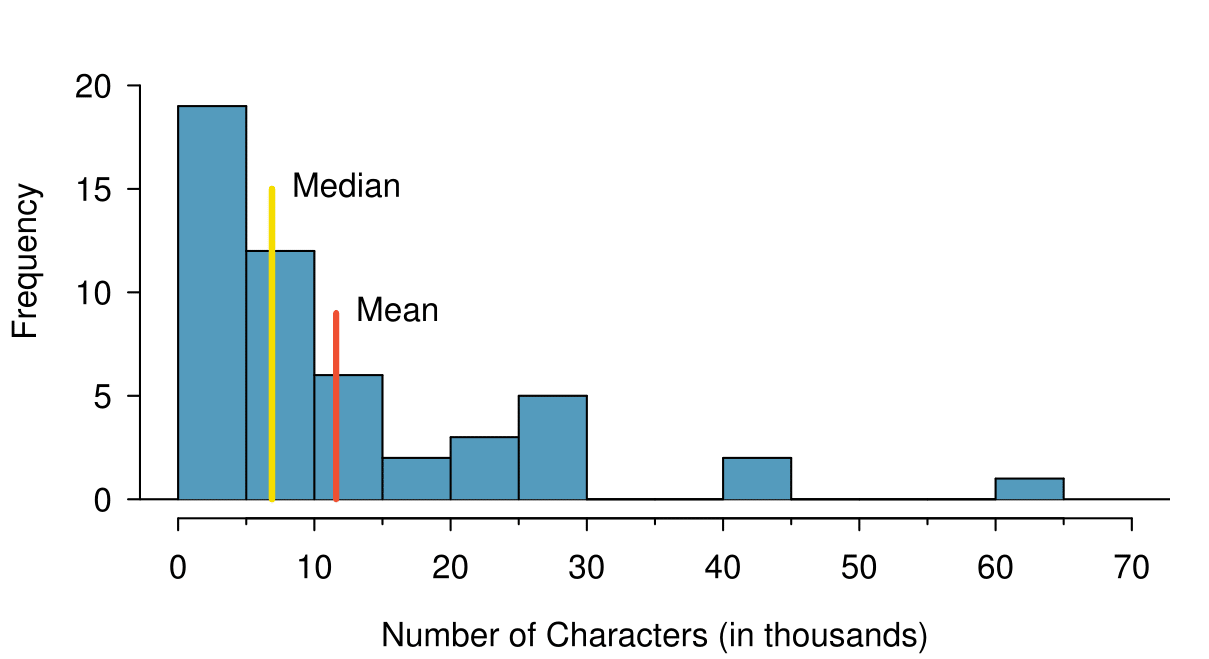Based on the data, why is the mean greater than the median in this data set?

Solution

Consider the three largest values of 42 thousand, 43 thousand, and 64 thousand. These values drag up the mean because they hstantially increase the sum (the total). However, they do not drag up the median because their magnitude does not change the location of the middle value.

###### The mean follows the tail

In a right skewed distribution, the mean is greater than the median.

In a left skewed distribution, the mean is less than the median.

In a symmetric distribution, the mean and median are approximately equal.

Consider the distribution of individual income in the United States. Which is greater: the mean or median? Why?  3 Because a small percent of individuals earn extremely large amounts of money while the majority earn a modest amount, the distribution is skewed to the right. Therefore, the mean is greater than the median.

### Subsection2.2.2Standard deviation as a measure of spread

The U.S. Census Bureau reported that in 2012, the median family income was $62,241 and the mean family income was$82,743. 4 www.census.gov/hhes/www/income/

Solution

###### TIP: comparing distributions

When comparing distributions, compare them with respect to center, spread, and shape as well as any unusual observations. Such descriptions should be in context.

What components of each plot in Figure 2.2.36 do you find most useful?  17 Answers will vary. The parallel box plots are especially useful for comparing centers and spreads, while the hollow histograms are more useful for seeing distribution shape, skew, and groups of anomalies.

Do these graphs tell us about any association between income for the two groups? 18 No, to see association we require a scatterplot. Moreover, these data are not paired, so the discussion of association does not make sense here.

Looking at an association is different than comparing distributions. When comparing distributions, we are interested in questions such as, “Which distribution has a greater average?” and “How do the shapes of the distribution differ?” The number of elements in each data set need not be the same (e.g. height of women and height of men). When we look at association, we are interested in whether there is a positive, negative, or no association between the variables. This requires two data sets of equal length that are essentially paired (e.g. height and weight of individuals).

###### TIP: comparing distributions versus looking at association

We compare two distributions with respect to center, spread, and shape. To compare the distributions visually, we use 2 single-variable graphs, such as two histograms, two dot plots, parallel box plots, or a back-to-back stem-and-leaf. When looking at association, we look for a positive, negative, or no relationship between the variables. To see association visually, we require a scatterplot.

### Subsection2.2.8Mapping data (special topic)

The county data set offers many numerical variables that we could plot using dot plots, scatterplots, or box plots, but these miss the true nature of the data. Rather, when we encounter geographic data, we should map it using an intensity map, where colors are used to show higher and lower values of a variable. Figure 2.2.40 and Figure 2.2.42 shows intensity maps for federal spending per capita (fed_spend), poverty rate in percent (poverty), homeownership rate in percent (homeownership), and median household income (med_income). The color key indicates which colors correspond to which values. Note that the intensity maps are not generally very helpful for getting precise values in any given county, but they are very helpful for seeing geographic trends and generating interesting research questions.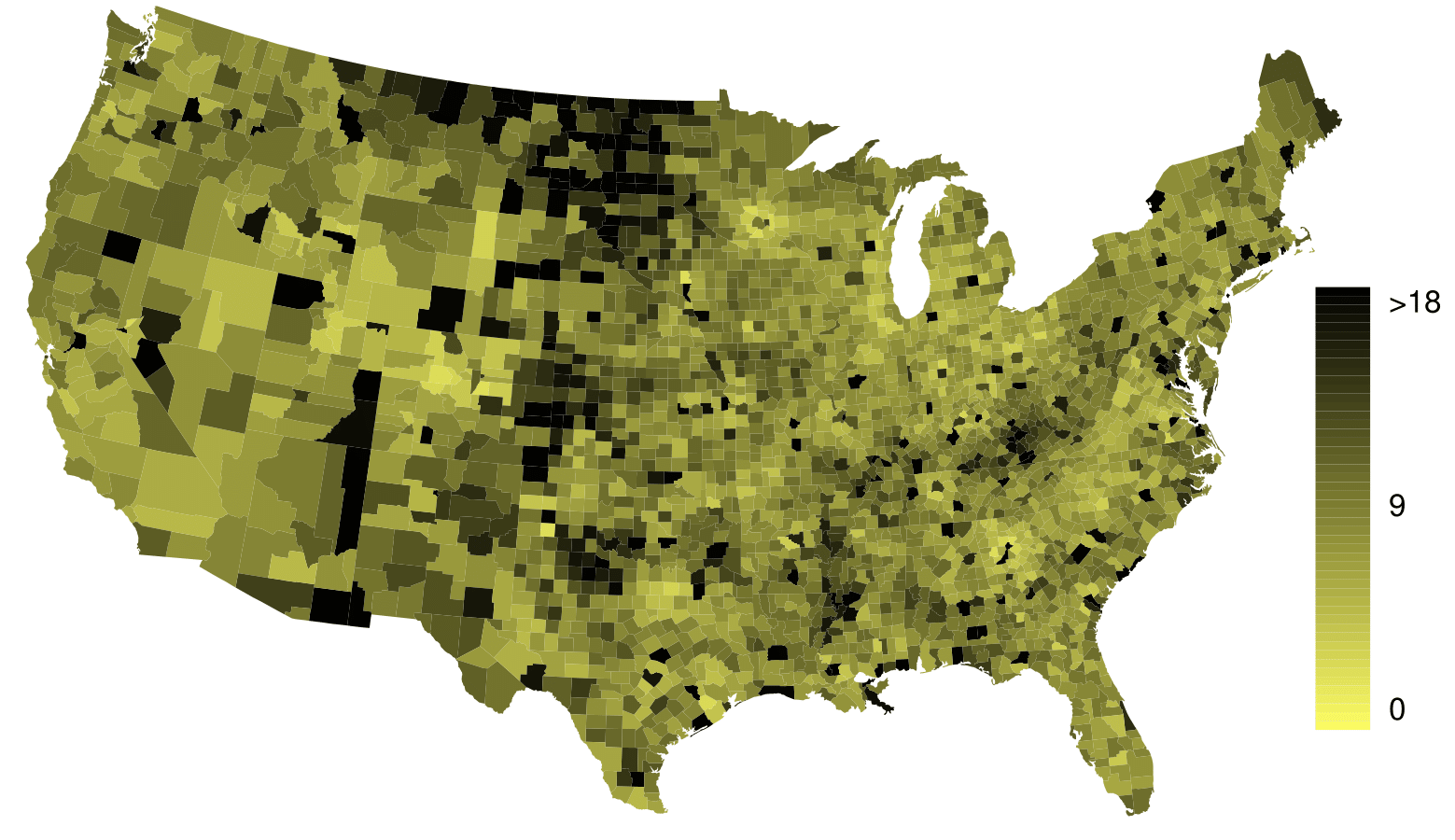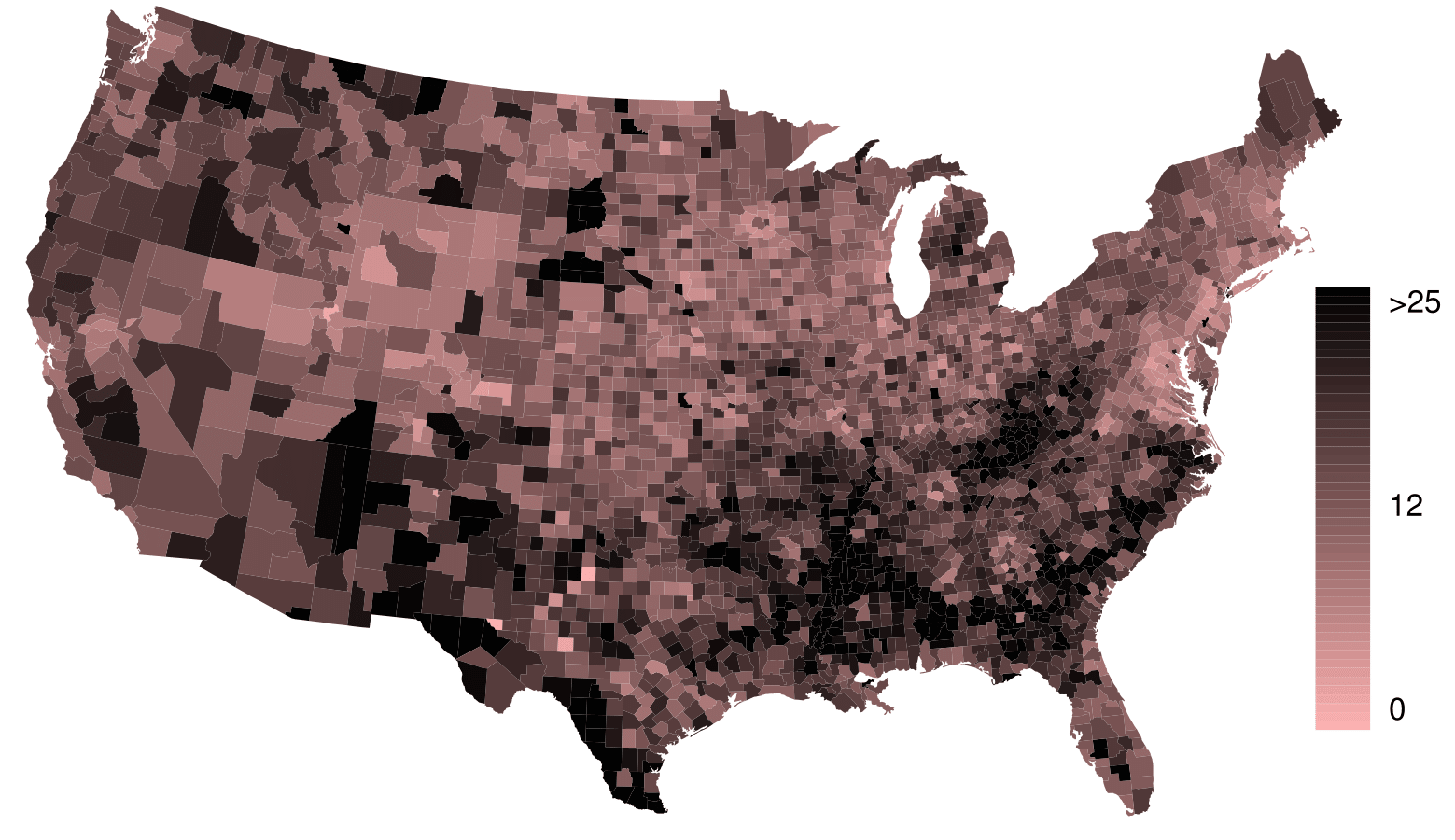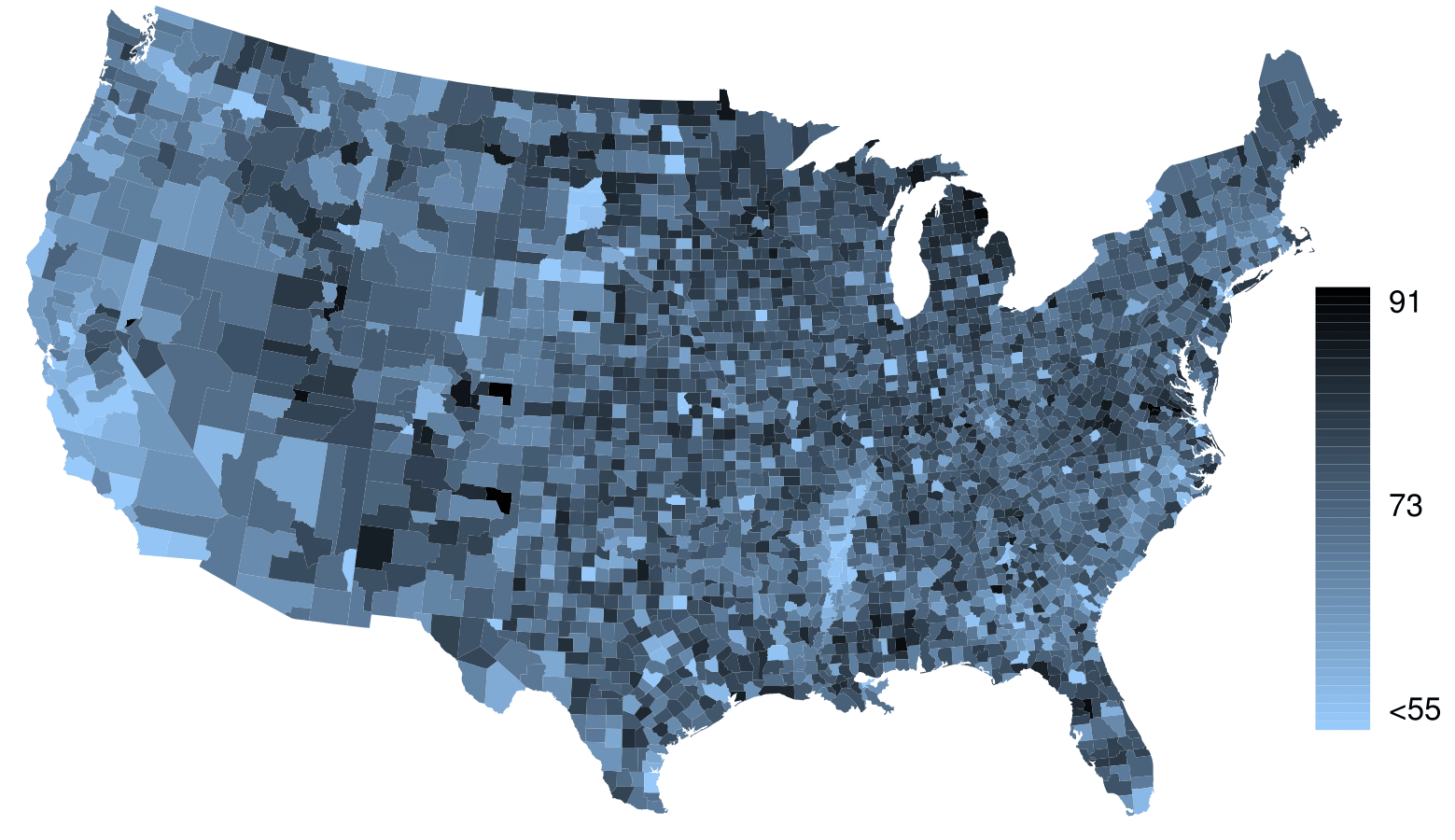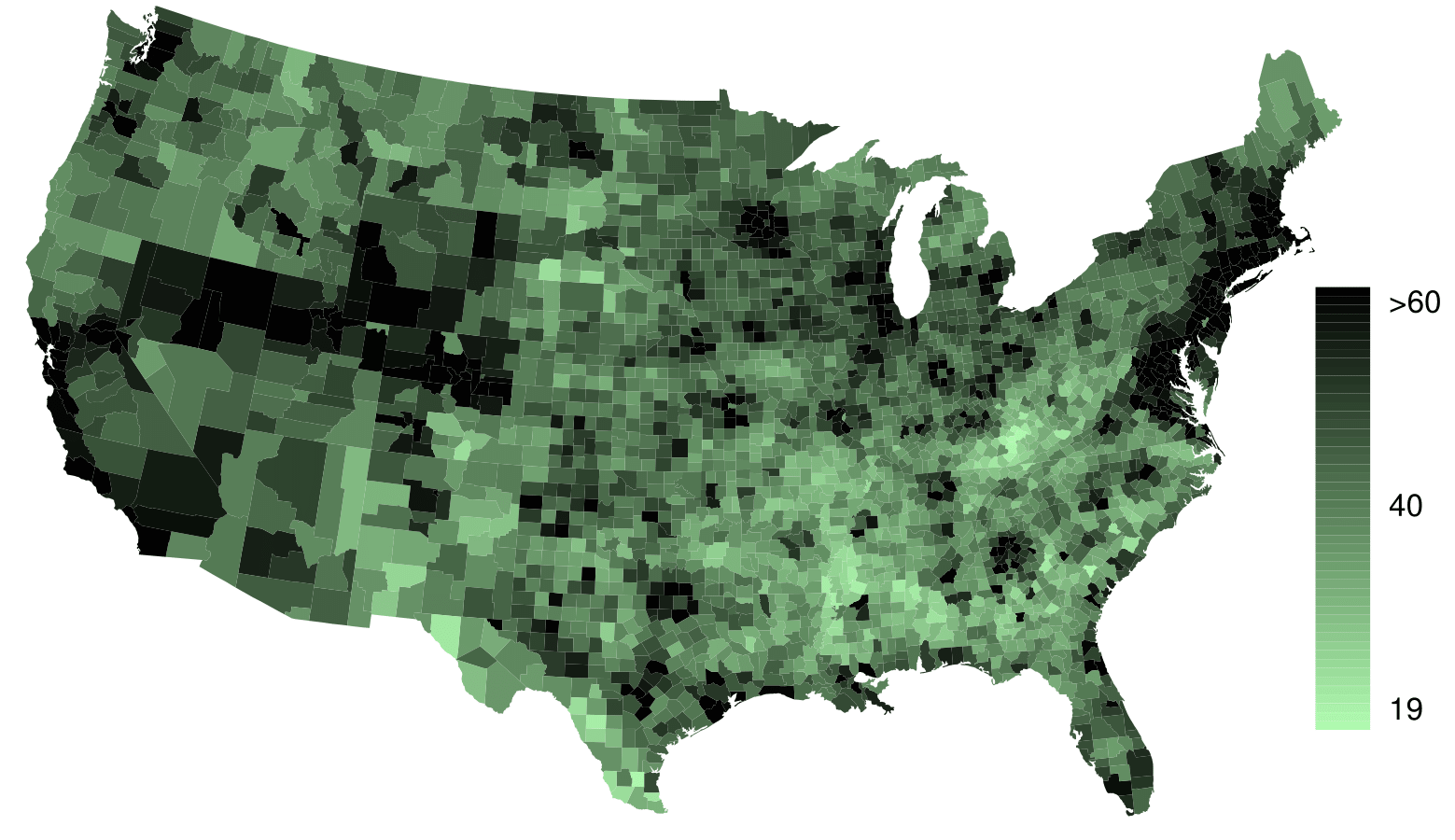What interesting features are evident in the fed_spend and poverty intensity maps?

Solution

The federal spending intensity map shows hstantial spending in the Dakotas and along the central-to-western part of the Canadian border, which may be related to the oil boom in this region. There are several other patches of federal spending, such as a vertical strip in eastern Utah and Arizona and the area where Colorado, Nebraska, and Kansas meet. There are also seemingly random counties with very high federal spending relative to their neighbors. If we did not cap the federal spending range at \$18 per capita, we would actually find that some counties have extremely high federal spending while there is almost no federal spending in the neighboring counties. These high-spending counties might contain military bases, companies with large government contracts, or other government facilities with many employees.

Poverty rates are evidently higher in a few locations. Notably, the deep south shows higher poverty rates, as does the southwest border of Texas. The vertical strip of eastern Utah and Arizona, noted above for its higher federal spending, also appears to have higher rates of poverty (though generally little correspondence is seen between the two variables). High poverty rates are evident in the Mississippi flood plains a little north of New Orleans and also in a large section of Kentucky and West Virginia.

What interesting features are evident in the med_income intensity map?  19 Note: answers will vary. There is a very strong correspondence between high earning and metropolitan areas. You might look for large cities you are familiar with and try to spot them on the map as dark spots.